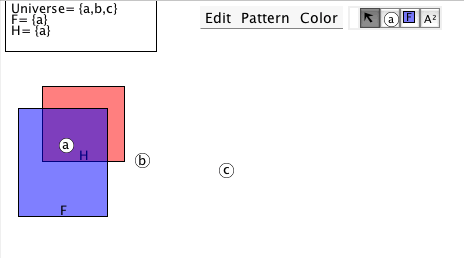# Tutorial 17: The semantics of quantifiers

Logical System

Under revision 2013

### The Tutorial

We will certainly wish to discuss the truth and falsity of formulas with quantifiers in them.

Interpretation 1

Universe= {a,b}
F={a}

and an atomic formula with a variable in it, Fx, say. Is this formula true in Interpretation 1? Well, the formula says 'x is F' and we know that x is supposed to be the name of something but the trouble is that we do not know what it is the name of. This makes quite a bit of difference-- if x is the name of the object a in the Universe then the formula is true, but if x is the name of the object b in the Universe then the formula is false. What has to insisted on here is some direction as to what x is supposed to name. x has to be valued. We have a notation for this, an example of it is

Fx[a/x]

and this is read 'Fx with x naming a' or 'Fx with x valued to be a'. Now, Fx[a/x] is true in Interpretation 1 whereas Fx[b/x] is false in Interpretation 1.

Our earlier work on Interpretations and Truth has to be extended to take in Valuations. What a Valuation should do is to assign to every variable in the language an entity in the Universe for that variable to denote. We have as variables m,n...z so a Valuation should give a value for all 14 of these. But, it is rare for a formula to contain more than a few variables; and whether a formula is true in a valuation depends only on the variables that the formula contains; so we can scrape by with valuing only the free variables that the formula contains. For example, take Gx... from a purist's point of view we should value every variable in the language, we should be looking at Gx[a/m,b/n,a/o,b/p,a/q,b/r,a/s,b/t,a/u,b/v,a/w,b/x,a/y,b/z] but Gx does not contain any of the variables m,n,o,p,q,r,s,t,u,v,w,y,z so we can squeek by with Gx[b/x].

A formula that has a Universal Quantifier as its main connective has its truth value assigned in an obvious way. Consider again

Interpretation 1 Universe= {a,b}
F={a}

and the formula

(∀x)(Fx).

The formula says 'whatever x you chose, x is F' and this is false in Interpretation 1 because if you chose b, b is not F, that is Fx[b/x] is false. (What you have to do mentally is to take the scope of the quantified formula and look also at the Universe of the Interpretation and run through Fx[a/x], Fx[b/x], ... for all the objects in the Universe and see if they are all suitable.)

A formula that has a Existential Quantifier as its main connective also has its truth value assigned in an obvious way. Consider again

Interpretation 1 Universe= {a,b}
F={a}

and the formula

(∃x)(Fx).

The formula says 'There is an x such that x is F' and this is true in Interpretation 1 because if you chose a, a is F, that is Fx[a/x] is true. (What you have to do mentally is to take the scope of the quantified formula and look also at the Universe of the Interpretation and run through Fx[a/x], Fx[b/x], ... for all the objects in the Universe and see if at least one will do the job.)

Some further concepts... If an occurrence of a variable occurs within the scope of a quantifier that uses that variable, that occurrence is said to be bound. Otherwise the occurrence is free. The need to value arises with formulas with free variables.

## Exercise to accompany Predicate Tutorial 7

The Interpretation in Predex7 shows an Interpretation similar to thisthe widget should display this, but if it does not just draw a similar one.

### Exercise 1 (of 3)

Some of the following formulas contain 'free variables'. Such formulas do not have truth values as they stand (for their truth depends on which individuals the free variables represent).

Formulas with free variables gain a truth value once their free variables are valued. And there is a notation for this. As an example, the formula Fx has the free variable x and in this form it lacks a truth value, but say we decide to let x stand for the individual a, using the notation Fx[a/x], then the formula thus interpreted has a truth value (it actually is true in this diagram).

Another example, the formula Fx[c/x] is false in the diagram. The notation [a/x] is read "a for x" so the formula Fx[c/x] is read "F of x with c for x".

### Exercise 3 (of 3).

If you decide to use the web application for the exercises you can launch it from here Deriver [Gentzen] — username 'logic' password 'logic'. Then either copy and paste the above formulas into the Journal or use the Deriver File Menu to Open Web Page with this address https://softoption.us/test/easyDeriver/CombinedExercisesEasyDGentzen.html .

Preferences

You may need to set some Preferences for this.

• you can check that the parser is set to gentzen.